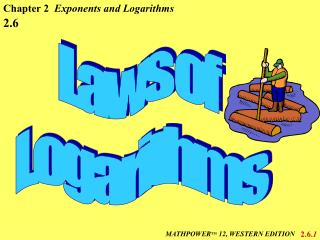Download PresentationLaws of Logarithms

# Laws of Logarithms

Download Presentation## Laws of Logarithms

- - - - - - - - - - - - - - - - - - - - - - - - - - - E N D - - - - - - - - - - - - - - - - - - - - - - - - - - -
##### Presentation Transcript

1. Chapter 2 Exponents and Logarithms 2.6 Laws of Logarithms 2.6.1 MATHPOWERTM 12, WESTERN EDITION

2. The Product and Quotient Laws Product Law: logb(mn) = logbm + logbn Quotient Law: as a sum and difference of logarithms: Express = log3A + log3B - log3C Evaluate: log210 + log212.8 = log2(10 x 12.8) = log2(128) = log2(27) = 7 2.6.2

3. Simplifying Logarithms Solve: x = log550 - log510 x = log55 = 1 Given that log79 = 1.129, find the value of log763: log763 = log7(9 x 7) = log79 + log77 = 1.129 + 1 = 2.129 Evaluate: x = log45a + log48a3 - log410a4 x = 1 x = log44 2.6.3

4. The Power Law Power Law: logbmn = n logbm Express as a single log: = log5216 2.6.4

5. Applying the Power Laws Evaluate: + 4(1) = 2(1) Given that log62 = 0.387 and log65 = 0.898 solve = 0.418 2.6.5

6. Applying the Power Laws Evaluate: = 4.135 If log28 = x, express each in terms of x: log28 = x log223 = x 3log22 = x b) log22 a) log2512 = log283 = 3log28 = 3x 2.6.6

7. Logarithm Applications The pH of a substance is defined by the equation pH = -log[H+], where H+ is the concentration of hydrogen ions in moles per litre (mol/L). Determine the pH if the hydrogen ion concentration is 0.000 012 5 mol/L. pH = -log[H+] = -log[1.25 x 10-5] = -(log1.25 + -5log10) = -(log1.25 - 5) = -(0.0969 - 5) = 4.9 Therefore, the pH is 4.9. Note: -log 0.000 012 5 = 4.9 2.6.7

8. Assignment Suggested Questions: Pages 106 and 107 1-18, 25, 31, 33-42, 47, 53 2.6.8